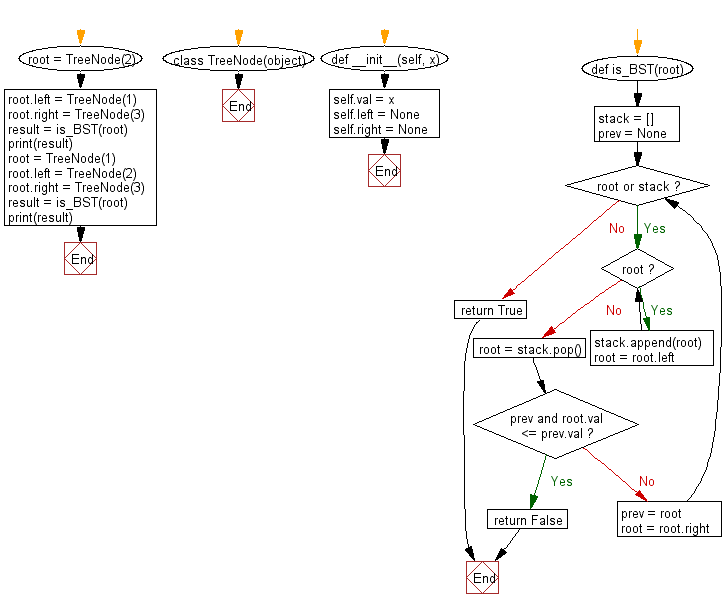﻿ Python Binary Search Tree (BST): Check a binary tree is valid or not- w3resource# Python Binary Search Tree: Check a binary tree is valid or not

## Python Binary Search Tree: Exercise-3 with Solution

Write a Python program to check whether a given a binary tree is a valid binary search tree (BST) or not.

Let a binary search tree (BST) is defined as follows:
The left subtree of a node contains only nodes with keys less than the node's key.
The right subtree of a node contains only nodes with keys greater than the node's key.
Both the left and right subtrees must also be binary search trees.

```Example 1:
2
/ \
1   3
Binary tree [2,1,3], return true.
Example 2:
1
/ \
2   3
Binary tree [1,2,3], return false.
```

Sample Solution:

Python Code:

``````class TreeNode(object):
def __init__(self, x):
self.val = x
self.left = None
self.right = None

def is_BST(root):
stack = []
prev = None

while root or stack:
while root:
stack.append(root)
root = root.left
root = stack.pop()
if prev and root.val <= prev.val:
return False
prev = root
root = root.right
return True

root = TreeNode(2)
root.left = TreeNode(1)
root.right = TreeNode(3)

result = is_BST(root)
print(result)

root = TreeNode(1)
root.left = TreeNode(2)
root.right = TreeNode(3)

result = is_BST(root)
print(result)
```
```

Sample Output:

```True
False
```

Flowchart:Python Code Editor:

What is the difficulty level of this exercise?

Test your Python skills with w3resource's quiz

﻿

## Python: Tips of the Day

Python: The Zip() Function

```>>> students = ('John', 'Mary', 'Mike')
>>> ages = (15, 17, 16)
>>> scores = (90, 88, 82, 17, 14)
>>> for student, age, score in zip(students, ages, scores):
...     print(f'{student}, age: {age}, score: {score}')
...
John, age: 15, score: 90
Mary, age: 17, score: 88
Mike, age: 16, score: 82
>>> zipped = zip(students, ages, scores)
>>> a, b, c = zip(*zipped)
>>> print(b)
(15, 17, 16)
```